# High Order Thinking Skills

## 20 Questions MCQ Test Mathematics Olympiad Class 4 | High Order Thinking Skills

Description
Attempt High Order Thinking Skills | 20 questions in 40 minutes | Mock test for Class 4 preparation | Free important questions MCQ to study Mathematics Olympiad Class 4 for Class 4 Exam | Download free PDF with solutions
QUESTION: 1

Solution:
QUESTION: 2

Solution:
QUESTION: 3

### Golu wants to move just one matchstick to make the equation correct. Which part will he take out ?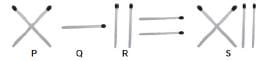Solution:
QUESTION: 4

Find the missing number in Fig. (X).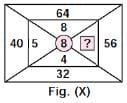Solution:
QUESTION: 5

The given bar graph shows the height of five children. What is the difference between the height of the tallest child and the shortest child ?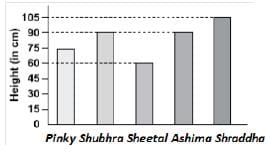Solution:
QUESTION: 6

If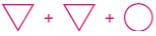= 120 and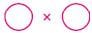=100, then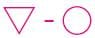= ?

Solution:

Circle x^2 = 100

x = 10

Triangle = 55

Hence 55 - 10 = 45

QUESTION: 7

Which of the following shows all the factors of 30?

Solution:
QUESTION: 8

Find the value of Y to make the expression true.
8000 = 50 × 80 × Y​

Solution:
QUESTION: 9

How much does the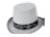cost?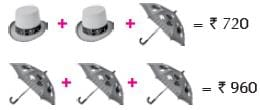Solution:
QUESTION: 10

Tom and Jerry sold together 120 chocolates during a sales promotion. Tom sold thrice as many chocolates than Jerry. How many chocolates did Jerry sell?

Solution:
QUESTION: 11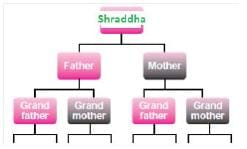Solution:
QUESTION: 12

Sheetal bought 120 peaches. She found out that out of every 10 peaches, 2 are rotten and had to be thrown away. How many peaches in the end are left with her?

Solution:

Out of 10, 2 peaches are rotton.

so out of 120, rotton peaches will be 120 x 2/10 = 24

so number of peaches left at the end are 120 - 24 = 96

QUESTION: 13

In a grazing field there are 50 goats, 40 deers and 10 children. How many legs are there in the field?

Solution:
QUESTION: 14

Which word CANNOT be made from the letters of the given word?EXPERIMENTAL

Solution:
QUESTION: 15

Complete the number pattern given below.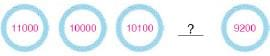Solution:
QUESTION: 16

If in a certain code ‘97452’ is written as ‘PRIME’, ‘503’ is written as ‘MUG’ and ‘6813’ is written as ‘TANG’, then how will ‘304687’ be written in the same code?

Solution:
QUESTION: 17

If the given matrix follows a certain rule row-wise or column-wise, then find the missing number.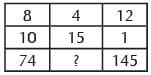Solution:
QUESTION: 18

There are _______ symmetrical figures and _______ non-symmetrical figures.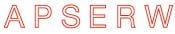Solution:

Symmetrical-A,E,W

non-symmetrical-P,S,R

QUESTION: 19

Shruti had Rs. 22500 in the bank. She put in another Rs. 2550. How much more money must she put in, if she wants to have a sum of ` 40000?

Solution:
QUESTION: 20

What is the weight of marble P?​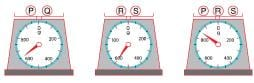Solution:Use Code STAYHOME200 and get INR 200 additional OFF Use Coupon Code